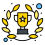# Bar Code for Products sold by weight## Recommended PostsCan you please tell if there is a specific number we need to add for the bar code?

In this guide some bar codes are. 14 digits and some are 13. https://help.loyverse.com/help/how-add-barcodes-items

It must be “20” or “02” for EAN 13 and “2” for UPC-A.

Please let know how to understand which one we should use EAN or UPC A.

WWWWW - weight (quantity) of item in grams. For example "01750" = 1.750kg

If I sell products in grammes does it have to be 00001 for 0.001 kg?

X - barcode checksum

Please explain what is X - barcode checksum.

Edited by Gleb_K
##### Share on other sitesIn this guide some bar codes are. 14 digits and some are 13. https://help.loyverse.com/help/how-add-barcodes-items

Please let know how many digits should it be?

##### Share on other sitesHello, dear user

You can use this tutorial only if you using the barcode label printing scales:

How to Scan Barcodes with Embedded Weight
https://help.loyverse.com/help/barcodes-with-embedded-weightYou can save any barcode in the item's settings. It will not affect the Embedded Weight barcode.
If you use the barcode label printing scales, the Embedded Weight barcode will be generated each time when you weight an item.
If you sell 1 gram of some product, you need to save it as "00001"  =  0.001 kg

X - barcode checksum:
A check digit, also known as a checksum character, is the number located on the far right side of a bar code. The purpose of a check digit is to verify that the information on the barcode has been entered correctly.

How to Scan Barcodes with Embedded Weight
https://help.loyverse.com/help/barcodes-with-embedded-weight

##### Share on other sitesThank you for your reply. The X - barcode checksum cannot be any random digit, right?

Could you tell how we can know this number. There are calculations to do it but I am nut sure if I do it right.

Would you have an information how to get this last number?

##### Share on other siteshttps://boxshot.com/barcode/tutorials/ean-13-calculator/

##### Share on other sitesWhen I calculate the bar code manually I gat one number. When I use the calculator it's another.

For example if my barcode is as below.

021010000001X

Where 02 is EAN13

10100 is the SKU

00001 is weight (as we sell by grams. It's soap to make it clear). Stands for 00 kg 001 gr

X is the check sum.

If I calculate manually following the guide from the link.

1. Sum all the digits in even positions and multiply by 3. I only have 2 multiplied by 3 = 6

2. Add all the the digits in odd positions (except for the last one which is check digit - I don't even have check digit yet. This is what I am trying to figure out. Why even to mention this. ) to the number you’ve got. OK. I got 3 (sum of all 1s)

3. Divide that number by 10 and take the reminder (I believe the number 3, right?) 3/10 = 0.3

4. If the reminder is not 0, subtract it from 10. 10-0.3 = 9.7

Obviously there is something wrong I am doing here or I understand it wrong.

If I put this number 021010000001 in the calculator it gives me 9 but I put 12 digits because I don't have the check number because this is what I am trying to calculate. The example in the calculator has 13 digits.

##### Share on other sitesHello

### Videos

×

• #### Partners

• App Marketplace